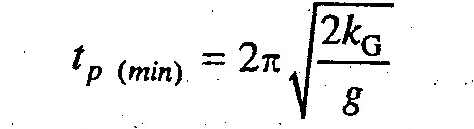# Compound Pendulum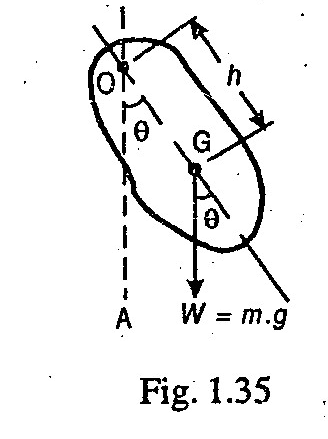When a rigid body is suspended vertically and it oscillates with a small amplitude under the action of force of gravity, the body is known as compound pendulum, as shown in Fig. 1.35.

For a compound pendulum, the periodic time is given by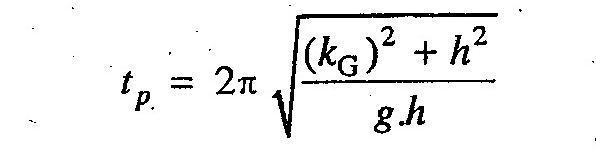and frequency of oscillation,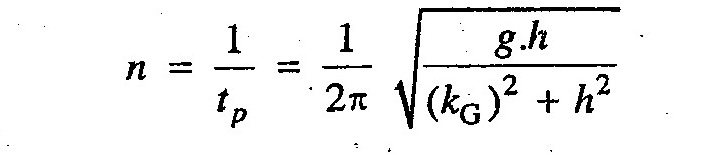where

kG = Radius of gyration about an axis through the center of gravity G and perpendicular to the plane of motion, and

h = Distance of point of suspension from the center of gravity G.

Notes:

1. The equivalent length of a simple pendulum (L) which gives the same frequency as that of compound pendulum is given by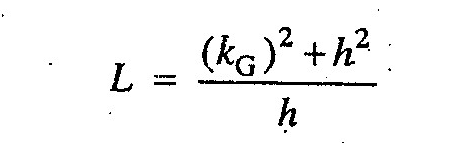2. The periodic time of compound pendulum is minimum when the distance between the point of suspension and the center of gravity (i.e. h) is equal to the radius of gyration of the body about its center of gravity (i.e. kG).

So, Minimum periodic time of a compound pendulum,